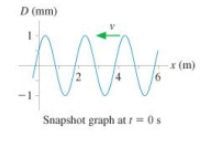# Problem: The figure is a snapshot graph at t = 0s of a 5.0 Hz wave traveling to the left.a) What is the wave speed?b) What is the phase constant of the wave?

###### FREE Expert Solution

a)

$\overline{){\mathbf{v}}{\mathbf{=}}{\mathbf{f}}{\mathbf{\lambda }}}$

f = 5.0 Hz

λ = 2 m

95% (195 ratings)###### Problem Details

The figure is a snapshot graph at t = 0s of a 5.0 Hz wave traveling to the left.

a) What is the wave speed?

b) What is the phase constant of the wave?